Courses

# Test: Arithmetic - 4 (2016-2014)

## 20 Questions MCQ Test UPSC Topic Wise Previous Year Questions | Test: Arithmetic - 4 (2016-2014)

Description
This mock test of Test: Arithmetic - 4 (2016-2014) for UPSC helps you for every UPSC entrance exam. This contains 20 Multiple Choice Questions for UPSC Test: Arithmetic - 4 (2016-2014) (mcq) to study with solutions a complete question bank. The solved questions answers in this Test: Arithmetic - 4 (2016-2014) quiz give you a good mix of easy questions and tough questions. UPSC students definitely take this Test: Arithmetic - 4 (2016-2014) exercise for a better result in the exam. You can find other Test: Arithmetic - 4 (2016-2014) extra questions, long questions & short questions for UPSC on EduRev as well by searching above.
QUESTION: 1

### In a race, a competitor has to collect 6 apples which are kept in a straight line on a track and a bucket is placed at the beginning of the track which is a starting point. The condition is that the competitor can pick only one apple at a time, run back with it and drop it in the bucket. If he has to drop all the apples in the bucket, how much total distance he has to run if the bucket is 5 meters from the first apple and all other apples are placed 3 meters apart? 

Solution: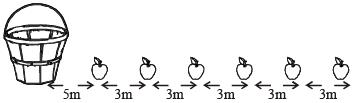Distances covered by the competitor to collect the apples in the bucket are as follows:
1st apple : 2(5) = 10 m
2nd apple : 2(5 + 3) = 16 m
3rd apple : 2(5 + 2 × 3) = 22 m
4th apple : 2(5 + 3 × 3) = 28 m
5th apple : 2(5 + 4 × 3) = 34 m
6th apple : 2(5 + 5 × 3) = 40 m
Therefore, total distance covered = 10 + 16 + 22 + 28 + 34 + 40 = 150 m

QUESTION: 2

### The average monthly income of a person in a certain family of 5 is ₹ 10,000. What will be the average monthly income of a person in the same family if the income of one person increased by ₹ 1,20,000 per year?  

Solution:

Average monthly income of a person in family of 5 = ₹ 10,000
∴ Monthly income of family = ₹  10,000 × 5 = ₹  50,000
After Increament
Increase in monthly income of one person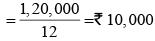Now, the average monthly income of a person in family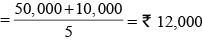QUESTION: 3

### W can do 25% of a work-in 30 days, X can do 1/4 of the work in 10 days, Y can do 40% of the work in 40 days and Z can do 1/3 of the work in 13 days. Who will complete the work first? 

Solution:

W can do 25% of a work in 30 days.
⇒ W can do 1/4 of a work in 30 days
∴  W can do the complete work in 120 days.
X can do 1/4  of the work in 10 days
∴  X can do the complete work in 40 days.
Y can do 40% of the work in 40 days.
⇒ Y can do 100% of the work in 100 days
∴ Y can do the complete work in 100 days.
Z can do 1/3 of the work in 13 days.
∴  Z can do the complete work in 39 days.
Hence, it is clear from above results that Z will complete the work first.

QUESTION: 4

How many numbers are there between 100 and 300 which either begin with or end with 2?



Solution:

From 100 to 199, there are 10 numbers ending with 2.
They are 102, 112, 122, 132, 142, 152, 162, 172, 182, 192.
And from 200 to 300, there are 100 numbers beginning with 2. They are 200, 201, 202...,299.
∴ There are 110 numbers between 100 and 300 which either begin with or end with 2.

QUESTION: 5

If R and S are different integers both divisible by 5, then which of the following is not necessarily true?



Solution:

By looking at all the options, we observe that option (b) is not necessarily true.
We know that, a number is divisible by 10 iff it has 0 at the unit’s place.
(R + S) may or may not have 0 at the unit’s place.
Therefore, it may or may not be divisible by 10.
Thus, it is not necessarily true.

QUESTION: 6

Ram and Shyam work on a job together for four days and complete 60% of it. Ram takes leave then and Shyam works for eight more days to complete the job. How long would Ram take to complete the entire job alone?



Solution:

Let the work done by Ram and Shyam be R and S respectively.
Given, (R+S)'s 4 days work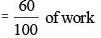⇒ (R + S)'s 1 day work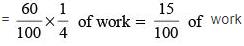After Ram takes leave :
S’s 8 days work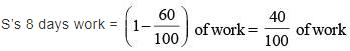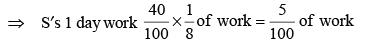So, Ram’s 1 day work = (R + S)'s 1 day work – S's 1 day work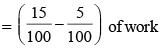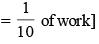∴ Ram will take 10 days to complete the entire job alone.

QUESTION: 7

AB is a vertical trunk of a huge tree with A being the point where the base of the trunk touches the ground. Due to a cyclone, the trunk has been broken at C which is at a height of 12 meters, broken part is partially attached to the vertical portion of the trunk at C. If the end of the broken part B touches the ground at D which is at a distance of 5 meters from A, then the original height of the trunk is:



Solution: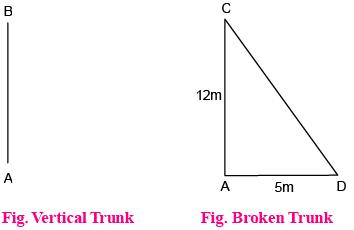According to pythagoras theorem,
CD2 = AD2 + AC2
⇒ CD2 = 52 + 122 ⇒ CD2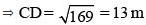Now, AB = AC + CD (∵ CD is the broken part of the trunk AB touching ground at D)
⇒ AB = (12 + 13) m = 25 m

QUESTION: 8

A person is standing on the first step from the bottom of a ladder. If he has to climb 4 more steps to reach exactly the middle step, how many steps does the ladder have?



Solution:

A person is standing on the first step from the bottom of a ladder.
Now, he has to climb 4 more steps to reach exactly the middle step,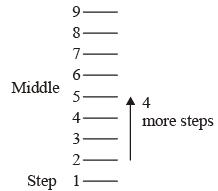So, it is clear that the ladder have 9 steps.

QUESTION: 9

The total emoluments of two persons are the same, but one gets allowances to the extent of 65% of his basic pay and the other gets allowances to the extent of 80% of his basic pay. The ratio of the basic pay of the former to the basic pay of the latter is:



Solution:

Let the basic pay of the first person be ₹ x and that of second person be ₹ y.
According to the question,
Emolument of both the persons are same.
So, x + 0.65x = y + 0.8y
⇒ x(1 + 0.65) = y(1 + 0.8)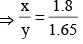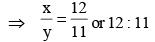QUESTION: 10

The sum of the ages of 5 members comprising a family, 3 years ago, was 80 years. The average age of the family today is the same as it was 3 years ago, because of an addition of a baby during the intervening period. How old is the baby?



Solution:

Let x1, x2, x3, x4 and x5 be the present ages of the family comprising of 5 members
3 years ago,
(x1 + x2 + x3 + x4 + x5) – 3 × 5 = 80
⇒ (x1 + x2 + x3 + x4 + x5) – 15 = 80 ...(i)
Now, let the age of new born baby be x6.
Given,
Average age of family today = Average age of family 3 years ago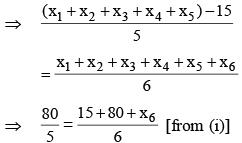⇒ x6 = 1
∴ Baby’s age = 1 year

QUESTION: 11

Candidates in a competitive examination consisted of 60% men and 40% women. 70% men and 75% women cleared the qualifying test and entered the final test where 80% men and 70% women were successful.
Which of the following statements is correct?



Solution:

Let initial population be100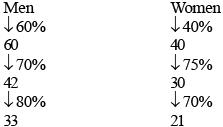It is clear that more men cleared the examination than women.

QUESTION: 12

A cow costs more than 4 goats but less than 5 goats. If a goat costs between ₹ 600 and ₹ 800, which of the following is a most valid conclusion?



Solution:

Let ‘g’ be the cost of goats.
4g < Cow < 5g
Now, Given a goat price = ₹ 600
2400 < Cow < 3000 ... (i)
Given, a goat price = ₹ 800
3200 < Cow < 4000 ... (ii)
Hence, it can be seen that from above equations that cow cost is between ₹ 2400 and ₹ 4000.

QUESTION: 13

The monthly incomes of Peter and Paul are in the ratio of 4 : 3. Their expenses are in the ratio of 3 : 2. If each saves ₹ 6,000 at the end of the month, their monthly incomes respectively are (in ₹)



Solution:

Let the Income of Peter and Paul are 4x and 3x
Let the Expenses of Peter and Paul are 3y and 2y
So, According to question
4x – 3y = 6000 ... (i)
3x – 2y = 6000 ... (ii)
Now, From equation (i) and equation (ii)
4x – 3y = 3x – 2y
∴ x = y ⇒ y = x
Now, put the value of y in equation (i), we get
⇒ x = 6000
∴ 4x = 24000
3x = 18000
Monthly Incomes of Peter and Paul are ₹ 24000 and ₹ 18000 respectively.

QUESTION: 14

Two equal glasses of same type are respectively 1/3 and 1/4 full of milk. They are then filled up with water and the contents are mixed in a pot. What is the ratio of milk and water in the pot?



Solution:

Let the capacity of each of the equal glass = x litre
Each glass containing x/3l and x/4l of milk.
The quantities of milk in first and the second glasses were x/3 and x/4 respectively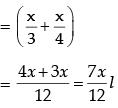So, the quantities of water in the first and the second glasses were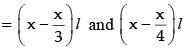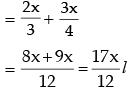Ratio of milk and water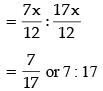QUESTION: 15

In a town, 45% population read magazine A, 55% read magazine B, 40% read magazine C, 30% read magazines A and B, 15% read magazines B and C, 25% read magazines A and C; and 10% read all the three magazines. What percentage do not read any magazine?



Solution: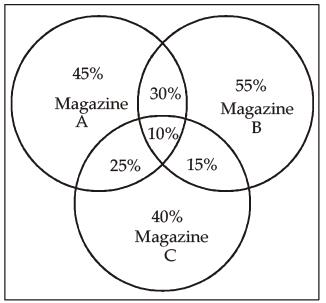Number of population who read the magazine
= (45 + 55 + 40) – (30 + 25 +15) + 10
= 140 – 70 + 10
= 80%
Number of population who do not read magazine = 100 – 80 = 20%

QUESTION: 16

As per agreement with a bank, a businessman had to refund a loan in some equal instalments without interest. After paying 18 instalments he found that 60 percent of his loan was refunded. How many instalments were there in the agreement?



Solution:

Let amount in each installment = x
Let total amount paid = L
According to Question.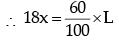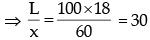= no. of installments.

QUESTION: 17

A bell rings every 18 minutes. A second bell rings every 24 minutes. A third bell rings every 32 minutes. If all the three bells ring at the same time at 8 o'clock in the morning, at what other time will they all ring together?



Solution:

LCM of 18, 24, 32
LCM of  9, 12, 16
LCM = 3 × 4 × 3 × 4
= 144 min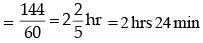∴ Bell will ring together again after 2 × (2 hrs 24 min) = 4 hrs. 48 min
i.e., 12 : 48 hrs

QUESTION: 18

Five persons fire bullets at a target at an interval of 6, 7, 8, 9 and 12 seconds respectively. The number of times they would fire the bullets together at the target in an hour is



Solution:

Time gap after which they will first hit the target is given by LCM of 6, 7, 8, 9, 12.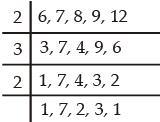LCM = (12 × 42) sec.
∴ In 1 hr (= 3600 sec) no. of time they will hit together is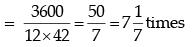QUESTION: 19

If Sohan, while selling two goats at the same price, makes a profit of 10% on one goat and suffers a loss of 10% on the other



Solution:

Let C.P of 1st goat is ₹ 100.
∴ S.P. of 1st goat is ₹110 (10% profit)
∴ S.P. of 2nd goat is ₹ 110 (Same S.P.)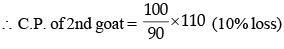= 1100/9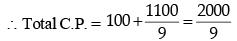Total S.P. = 2 × 110 = 220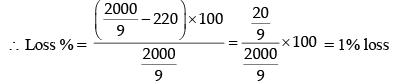Shortcut method: Loss %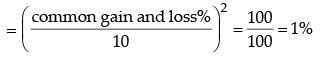QUESTION: 20

For a charity show, the total tickets sold were 420. Half of these tickets were sold at the rate of ₹ 5 each, one-third at the rate of ₹ 3 each and the rest for ₹ 2 each. What was the total amount received?



Solution:

Total amount received is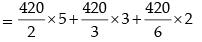= 210 × 5 + 140 × 3 + 70 × 2
= 1050 + 420 + 140 = ₹ 1610